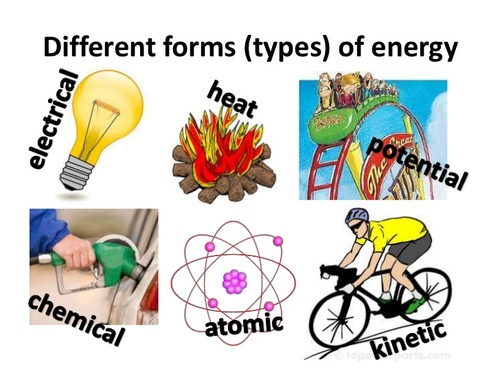Forms of Energy
3 years ago
rblayne765
Save
Edit
Host a game
Live GameLive
Homework
Solo Practice
Practice12 QuestionsShow answers
• Question 1
20 secondsQ. Energy stored in the bonds between atoms
chemical energy
mechanical energy
kinetic energy
nuclear energy
• Question 2
20 secondsQ. Energy stored in an atom's nucleus
chemical energy
mechanical energy
kinetic energy
nuclear energy
• Question 3
20 secondsQ. Energy stored in a flexed or compressed object
gravitational potential energy
mechanical energy
kinetic energy
elastic potential energy
• Question 4
20 secondsQ. Energy of motion
gravitational potential energy
mechanical energy
kinetic energy
elastic potential energy
• Question 5
20 secondsQ. Potential energy due to gravity
gravitational potential energy
mechanical energy
kinetic energy
elastic potential energy
• Question 6
20 secondsQ. Energy in the form of waves that can move through space
gravitational potential energy
electromagnetic energy
sound energy
elastic potential energy
• Question 7
20 secondsQ. Energy in the form of waves that move through a medium like air or water
gravitational potential energy
electromagnetic energy
sound energy
elastic potential energy
• Question 8
20 secondsQ. Energy of moving electrons
electrical energy
electromagnetic energy
sound energy
mechanical energy
• Question 9
20 secondsQ. Energy of vibrating particles
electrical energy
thermal energy
sound energy
mechanical energy
• Question 10
20 secondsQ. Kinetic energy + potential energy
electrical energy
thermal energy
sound energy
mechanical energy
• Question 11
20 seconds
Q. Stored energy
electrical energy
kinetic energy
potential energy
mechanical energy
• Question 12
20 seconds
Q. The ability to do work